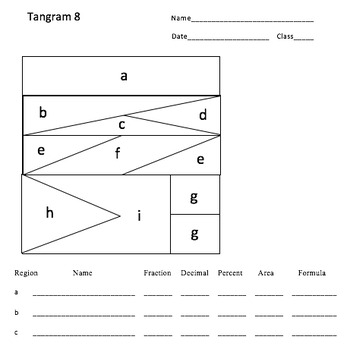# Tangram Math: Integrating Fractions, Decimals, Percent, Geometry, and Algebra

Rated 5 out of 5, based on 4 reviews
4 Ratings;
5th - 8th, Homeschool
Subjects
Resource Type
Standards
Formats Included
• PDF
Pages
23 pages
Report this resource to TPT

#### What educators are saying

This product looks great on first glance as I am reviewing the concept and planning lessons! I'll update the review when I have used this with students. THANK YOU!
My students were so engaged by this activity! They were extremely eager to solve as many of these as possible!

### Description

In this powerfully engaging activity students of all skill levels will study standard and nonstandard tangrams to determine the values of the pieces. Students will compare the pieces to see how they relate to one another as fractions, decimals, percent, and areas. They will also develop geometric vocabulary and form an understanding of congruence and similarity. The best part is that the entire process will lead them seamlessly into algebraic thinking as they navigate among the pieces and their representations. The activity can also be used to help students learn probability, solve proportions and equations and understand algebraic properties!

Total Pages
23 pages
Included
Teaching Duration
1 Week
Report this resource to TPT
Reported resources will be reviewed by our team. Report this resource to let us know if this resource violates TPT’s content guidelines.

### Standards

to see state-specific standards (only available in the US).
Read, write, and compare decimals to thousandths.
Read and write decimals to thousandths using base-ten numerals, number names, and expanded form, e.g., 347.392 = 3 × 100 + 4 × 10 + 7 × 1 + 3 × (1/10) + 9 × (1/100) + 2 × (1/1000).
Compare two decimals to thousandths based on meanings of the digits in each place, using >, =, and < symbols to record the results of comparisons.
Add and subtract fractions with unlike denominators (including mixed numbers) by replacing given fractions with equivalent fractions in such a way as to produce an equivalent sum or difference of fractions with like denominators. For example, 2/3 + 5/4 = 8/12 + 15/12 = 23/12. (In general, 𝘢/𝘣 + 𝘤/𝘥 = (𝘢𝘥 + 𝘣𝘤)/𝘣𝘥.)
Solve word problems involving addition and subtraction of fractions referring to the same whole, including cases of unlike denominators, e.g., by using visual fraction models or equations to represent the problem. Use benchmark fractions and number sense of fractions to estimate mentally and assess the reasonableness of answers. For example, recognize an incorrect result 2/5 + 1/2 = 3/7, by observing that 3/7 < 1/2.